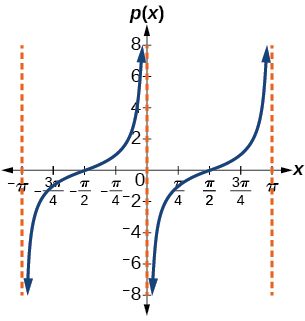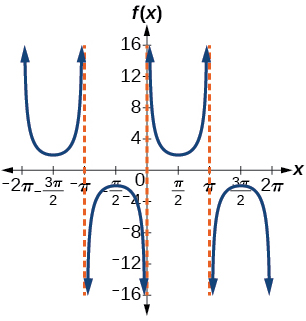$$\newcommand{\id}{\mathrm{id}}$$ $$\newcommand{\Span}{\mathrm{span}}$$ $$\newcommand{\kernel}{\mathrm{null}\,}$$ $$\newcommand{\range}{\mathrm{range}\,}$$ $$\newcommand{\RealPart}{\mathrm{Re}}$$ $$\newcommand{\ImaginaryPart}{\mathrm{Im}}$$ $$\newcommand{\Argument}{\mathrm{Arg}}$$ $$\newcommand{\norm}{\| #1 \|}$$ $$\newcommand{\inner}{\langle #1, #2 \rangle}$$ $$\newcommand{\Span}{\mathrm{span}}$$

# 6.E: Periodic Functions (Exercises)

$$\newcommand{\vecs}{\overset { \rightharpoonup} {\mathbf{#1}} }$$ $$\newcommand{\vecd}{\overset{-\!-\!\rightharpoonup}{\vphantom{a}\smash {#1}}}$$$$\newcommand{\id}{\mathrm{id}}$$ $$\newcommand{\Span}{\mathrm{span}}$$ $$\newcommand{\kernel}{\mathrm{null}\,}$$ $$\newcommand{\range}{\mathrm{range}\,}$$ $$\newcommand{\RealPart}{\mathrm{Re}}$$ $$\newcommand{\ImaginaryPart}{\mathrm{Im}}$$ $$\newcommand{\Argument}{\mathrm{Arg}}$$ $$\newcommand{\norm}{\| #1 \|}$$ $$\newcommand{\inner}{\langle #1, #2 \rangle}$$ $$\newcommand{\Span}{\mathrm{span}}$$ $$\newcommand{\id}{\mathrm{id}}$$ $$\newcommand{\Span}{\mathrm{span}}$$ $$\newcommand{\kernel}{\mathrm{null}\,}$$ $$\newcommand{\range}{\mathrm{range}\,}$$ $$\newcommand{\RealPart}{\mathrm{Re}}$$ $$\newcommand{\ImaginaryPart}{\mathrm{Im}}$$ $$\newcommand{\Argument}{\mathrm{Arg}}$$ $$\newcommand{\norm}{\| #1 \|}$$ $$\newcommand{\inner}{\langle #1, #2 \rangle}$$ $$\newcommand{\Span}{\mathrm{span}}$$

## 6.1: Graphs of the Sine and Cosine Functions

In the chapter on Trigonometric Functions, we examined trigonometric functions such as the sine function. In this section, we will interpret and create graphs of sine and cosine functions

### Verbal

1) Why are the sine and cosine functions called periodic functions?

The sine and cosine functions have the property that $$f(x+P)=f(x)$$ for a certain $$P$$. This means that the function values repeat for every $$P$$ units on the $$x$$-axis.

2) How does the graph of $$y=\sin x$$ compare with the graph of $$y=\cos x$$? Explain how you could horizontally translate the graph of $$y=\sin x$$ to obtain $$y=\cos x$$.

3) For the equation $$A \cos(Bx+C)+D$$$,$what constants affect the range of the function and how do they affect the range?

The absolute value of the constant $$A$$ (amplitude) increases the total range and the constant $$D$$ (vertical shift) shifts the graph vertically.

4) How does the range of a translated sine function relate to the equation $$y=A \sin(Bx+C)+D$$$?$

5) How can the unit circle be used to construct the graph of $$f(t)=\sin t$$?

At the point where the terminal side of $$t$$ intersects the unit circle, you can determine that the $$\sin t$$ equals the $$y$$-coordinate of the point.

### Graphical

For the following exercises, graph two full periods of each function and state the amplitude, period, and midline. State the maximum and minimum $$y$$-values and their corresponding $$x$$-values on one period for $$x>0$$. Round answers to two decimal places if necessary.

6) $$f(x)=2\sin x$$

7) $$f(x)=\dfrac{2}{3}\cos x$$amplitude: $$\dfrac{2}{3}$$$;$period: $$2\pi$$$;$midline: $$y=0$$$;$maximum: $$y=\dfrac{2}{3}$$ occurs at $$x=0$$$;$minimum: $$y=-\dfrac{2}{3}$$ occurs at $$x=\pi$$$;$for one period, the graph starts at $$0$$ and ends at $$2\pi$$.

8) $$f(x)=-3\sin x$$

9) $$f(x)=4\sin x$$amplitude: $$4$$; period: $$2\pi$$$;$midline: $$y=0$$$;$maximum $$y=4$$ occurs at $$x=\dfrac{\pi }{2}$$$;$minimum: $$y=-4$$ occurs at $$x=\dfrac{3\pi }{2}$$$;$one full period occurs from $$x=0$$ to $$x=2\pi$$

10) $$f(x)=2\cos x$$

11) $$f(x)=\cos (2x)$$amplitude: $$1$$; period: $$\pi$$$;$midline:

## 6.2: Graphs of the Other Trigonometric Functions

### Graphical## 6.3: Inverse Trigonometric Functions

In this section, we will explore the inverse trigonometric functions. Inverse trigonometric functions “undoes” what the original trigonometric function “does,” as is the case with any other function and its inverse. In other words, the domain of the inverse function is the range of the original function, and vice versa.

### Verbal

1) Why do the functions $$f(x)=\sin^{-1}x$$ and $$g(x)=\cos^{-1}x$$ have different ranges?

The function $$y=\sin x$$ is one-to-one on $$\left [ -\dfrac{\pi }{2},\dfrac{\pi }{2} \right ]$$$;$ thus, this interval is the range of the inverse function of $$y=\sin x$$, $$f(x)=\sin^{-1}x$$ The function $$y=\cos x$$ is one-to-one on $$[0,\pi ]$$$;$ thus, this interval is the range of the inverse function of $$y=\cos x$$, $$f(x)=\cos^{-1}x$$

2) Since the functions $$y=\cos x$$ and $$y=\cos^{-1}x$$ are inverse functions, why is $$\cos^{-1}\left (\cos \left (-\dfrac{\pi }{6} \right ) \right )$$not equal to $$-\dfrac{\pi }{6}$$?

3) Explain the meaning of $$\dfrac{\pi }{6}=\arcsin (0.5)$$.

$$\dfrac{\pi }{6}$$ is the radian measure of an angle between $$-\dfrac{\pi }{2}$$ and $$\dfrac{\pi }{2}$$ whose sine is $$0.5$$.

4) Most calculators do not have a key to evaluate $$\sec ^{-1}(2)$$$.$Explain how this can be done using the cosine function or the inverse cosine function.

5) Why must the domain of the sine function, $$\sin x$$$,$be restricted to $$\left [ -\dfrac{\pi }{2},\dfrac{\pi }{2} \right ]$$ for the inverse sine function to exist?

In order for any function to have an inverse, the function must be one-to-one and must pass the horizontal line test. The regular sine function is not one-to-one unless its domain is restricted in some way. Mathematicians have agreed to restrict the sine function to the interval $$\left [ -\dfrac{\pi }{2},\dfrac{\pi }{2} \right ]$$ so that it is one-to-one and possesses an inverse.

6) Discuss why this statement is incorrect: $$\arccos(\cos x)=x$$ for all $$x$$.

7) Determine whether the following statement is true or false and explain your answer: $$\arccos(-x)=\pi - \arccos x$$

True . The angle, $$\theta _1$$ that equals $$\arccos(-x)$$, $$x>0$$, will be a second quadrant angle with reference angle, $$\theta _2$$, where $$\theta _2$$ equals $$\arccos x$$, $$x>0$$. Since $$\theta _2$$ is the reference angle for $$\theta _1$$, $$\theta _2=\pi - \theta _1$$ and $$\arccos(-x)=\pi - \arccos x-$$

### Algebraic

For the exercises 8-16, evaluate the expressions.

8) $$\sin^{-1}\left(\dfrac{\sqrt{2}}{2}\right)$$

9) $$\sin^{-1}\left(-\dfrac{1}{2}\right)$$

$$-\dfrac{\pi }{6}$$

10) $$\cos^{-1}\left(-\dfrac{1}{2}\right)$$

11) $$\cos^{-1}\left(-\dfrac{\sqrt{2}}{2}\right)$$

$$\dfrac{3\pi }{4}$$

12) $$\tan^{-1}(1)$$

13) $$\tan^{-1}(-\sqrt{3})$$

$$-\dfrac{\pi }{3}$$

14) $$\tan^{-1}(-1)$$

15) $$\tan^{-1}(\sqrt{3})$$

$$\dfrac{\pi }{3}$$

16) $$\tan^{-1}\left(\dfrac{-1}{\sqrt{3}}\right)$$

For the exercises 17-21, use a calculator to evaluate each expression. Express answers to the nearest hundredth.

17) $$\cos^{-1}(-0.4)$$

$$1.98$$

18) $$\arcsin (0.23)$$

19) $$\arccos \left(\dfrac{3}{5}\right)$$

$$0.93$$

20) $$\cos^{-1}(-0.8)$$

21) $$\tan^{-1}(6)$$

$$1.41$$

For the exercises 22-23, find the angle $$\theta$$ in the given right triangle. Round answers to the nearest hundredth.

22)23)$$0.56$$ radians

For the exercises 24-36, find the exact value, if possible, without a calculator. If it is not possible, explain why.

24) $$\sin^{-1}(\cos(\pi))$$

25) $$\tan^{-1}(\sin(\pi))$$

$$0$$

26) $$\cos^{-1}\left(\sin \left(\dfrac{\pi}{3} \right)\right)$$

27) $$\tan^{-1}\left(\sin \left(\dfrac{\pi}{3} \right)\right)$$

$$0.71$$

28) $$\sin^{-1}\left(\cos \left(\dfrac{-\pi}{2} \right)\right)$$

29) $$\tan^{-1}\left(\sin \left(\dfrac{4\pi}{3} \right)\right)$$

$$-0.71$$

30) $$\sin^{-1}\left(\sin \left(\dfrac{5\pi}{6} \right)\right)$$

31) $$\tan^{-1}\left(\sin \left(\dfrac{-5\pi}{2} \right)\right)$$

$$-\dfrac{\pi}{4}$$

32) $$\cos \left(\sin^{-1} \left(\dfrac{4}{5} \right)\right)$$

33) $$\sin \left(\cos^{-1} \left(\dfrac{3}{5} \right)\right)$$

$$0.8$$

34) $$\sin \left(\tan^{-1} \left(\dfrac{4}{3} \right)\right)$$

35) $$\cos \left(\tan^{-1} \left(\dfrac{12}{5} \right)\right)$$

$$\dfrac{5}{13}$$

36) $$\cos \left(\sin^{-1} \left(\dfrac{1}{2} \right)\right)$$

For the exercises 37-41, find the exact value of the expression in terms of $$x$$ with the help of a reference triangle.

37) $$\tan \left(\sin^{-1} (x-1)\right)$$

$$\dfrac{x-1}{\sqrt{-x^2+2x}}$$

38) $$\sin \left(\sin^{-1} (1-x)\right)$$

39) $$\cos \left(\sin^{-1} \left(\dfrac{1}{x}\right)\right)$$

$$\dfrac{\sqrt{x^2-1}}{x}$$

40) $$\cos \left(\tan^{-1} (3x-1)\right)$$

41) $$\tan \left(\sin^{-1} \left(x+\dfrac{1}{2}\right)\right)$$

$$\dfrac{x+0.5}{\sqrt{-x^2-x+\tfrac{3}{4}}}$$

### Extensions

For the exercise 42, evaluate the expression without using a calculator. Give the exact value.

2) $$\dfrac{\sin^{-1}\left ( \tfrac{1}{2} \right )-\cos^{-1}\left ( \tfrac{\sqrt{2}}{2} \right )+\sin^{-1}\left ( \tfrac{\sqrt{3}}{2} \right )-\cos^{-1}(1)}{\cos^{-1}\left ( \tfrac{\sqrt{3}}{2} \right )-\sin^{-1}\left ( \tfrac{\sqrt{2}}{2} \right )+\cos^{-1}\left ( \tfrac{1}{2} \right )-\sin^{-1}(0)}$$

For the exercises 43-47, find the function if $$\sin t = \dfrac{x}{x+1}$$

43) $$\cos t$$

$$\dfrac{\sqrt{2x+1}}{x+1}$$

44) $$\sec t$$

45) $$\cot t$$

$$\dfrac{\sqrt{2x+1}}{x}$$

46) $$\cos \left(\sin^{-1} \left(\dfrac{x}{x+1}\right)\right)$$

47) $$\tan^{-1} \left(\dfrac{x}{\sqrt{2x+1}}\right)$$

$$t$$

### Graphical

48) Graph $$y=\sin^{-1} x$$ and state the domain and range of the function.

49) Graph $$y=\arccos x$$ and state the domain and range of the function.domain $$[-1,1]$$$;$range $$[0,\pi ]$$

50) Graph one cycle of $$y=\tan^{-1} x$$ and state the domain and range of the function.

51) For what value of $$x$$ does $$\sin x=\sin^{-1} x$$? Use a graphing calculator to approximate the answer.

approximately $$x=0.00$$

52) For what value of $$x$$ does $$\cos x=\cos^{-1} x$$? Use a graphing calculator to approximate the answer.

### Real-World Applications

53) Suppose a $$13$$-foot ladder is leaning against a building, reaching to the bottom of a second-ﬂoor window $$12$$ feet above the ground. What angle, in radians, does the ladder make with the building?

$$0.395$$ radians

54) Suppose you drive $$0.6$$ miles on a road so that the vertical distance changes from $$0$$ to $$150$$ feet. What is the angle of elevation of the road?

55) An isosceles triangle has two congruent sides of length $$9$$ inches. The remaining side has a length of $$8$$ inches. Find the angle that a side of $$9$$ inches makes with the $$8$$-inch side.

$$1.11$$ radians

56) Without using a calculator, approximate the value of $$\arctan (10,000)$$$.$Explain why your answer is reasonable.

57) A truss for the roof of a house is constructed from two identical right triangles. Each has a base of $$12$$ feet and height of $$4$$ feet. Find the measure of the acute angle adjacent to the $$4$$-foot side.

$$1.25$$ radians

58) The line $$y=\dfrac{3}{5}x$$ passes through the origin in the $$x,y$$-plane. What is the measure of the angle that the line makes with the positive $$x$$-axis?

59) The line $$y=\dfrac{-3}{7}x$$ passes through the origin in the $$x,y$$-plane. What is the measure of the angle that the line makes with the negative $$x$$-axis?

$$0.405$$ radians

60) What percentage grade should a road have if the angle of elevation of the road is $$4$$ degrees? (The percentage grade is defined as the change in the altitude of the road over a $$100$$-foot horizontal distance. For example a $$5\%$$ grade means that the road rises $$5$$ feet for every $$100$$ feet of horizontal distance.)

61) A $$20$$-foot ladder leans up against the side of a building so that the foot of the ladder is $$10$$ feet from the base of the building. If specifications call for the ladder's angle of elevation to be between $$35$$ and $$45$$ degrees, does the placement of this ladder satisfy safety specifications?

No. The angle the ladder makes with the horizontal is $$60$$ degrees.

62) Suppose a $$15$$-foot ladder leans against the side of a house so that the angle of elevation of the ladder is $$42$$ degrees. How far is the foot of the ladder from the side of the house?